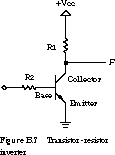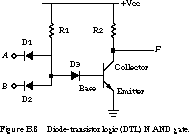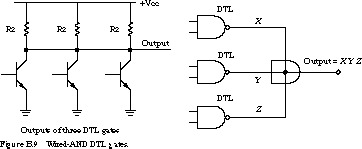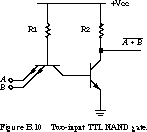[Top] [Next] [Prev]

B.3 Bipolar Transistor Logic

In this subsection, we examine how to build logic gates from bipolar transistors, the dominant technology of the 1970s and early 1980s.

B.3.1 Basic Bipolar Transistor Logic

A bipolar transistor is a three-terminal semiconductor device. Under the control of one of the terminals, called the base, current can flow selectively from the collector terminal to the emitter terminal. Using transistors as electronically controlled switches is critical for building modern digital logic.

Using our water analogy, a transistor is like a water spigot. The base is like a screw. When it is tightened down, no water (current) flows through the spigot. The transistor is in its "off" region of operation. As the screw is turned, the flow of water begins to trickle and then increases. This is the so-called linear operating region. The current increases linearly as the voltage across the transistor is increased. Eventually, the flow reaches a point where opening the screw further does not increase the flow, it being limited by the diameter of the pipe. This is called the saturation region. Despite changes in voltage across the transistors, there is no change in current. Transistors in digital logic pass quickly from the off region to the saturation region.

The basic inverter constructed from transistors and resistors is shown in Figure B.7 (for those in the know, the transistor shown is an NPN transistor).A high voltage at the base turns on the transistor. The output F is discharged to ground, getting close to 0 V but never quite reaching it (it reaches a voltage drop away from 0 V).

When a low voltage is placed on the base, the transistor is turned off. The output node F is charged up toward the power supply voltage through the pull-up/load resistor R1.

B.3.2 Diode-Transistor Logic

Diodes, transistors, and resistors can be used to implemented a wide variety of gates. Basically, we combine the diode logic of Figure B.6 with the transistor inverter of Figure B.7 to form NAND (not AND) and NOR (not OR) functions.

A two-input DTL (diode-transistor logic) NAND gate is shown in Figure B.8.It works like this. The diodes marked D1 and D2, together with resistor R1, form a two-input AND function. At the input to D3, a logic 0 is represented by approximately 0.7 V, while a logic 1 is in the range 4 to 5 V. D3 increases the voltage required to turn on the transistor. This gives a better separation between the voltage levels recognized as a logic 0 and logic 1.

For the transistor to conduct, D3 must be turned on. This happens when the anode voltage reaches 1.4 V. If the voltage at the anode is much higher than this, the base will be driven to a high voltage. The transistor will be strongly turned on, with low resistance, and thus F will be discharged toward 0 V. If the anode is at a low voltage, the base will also be low. This keeps the transistor off (essentially infinite resistance), allowing the output node to reach a logic 1 voltage level.

Gates constructed as in Figure B.8 have a limit to the number of gate inputs to which their output can be connected. This is called fan-out. The pull-up resistor R2 is what limits the fan-out. The output F is at Vcc as long as no current is being drawn from the power supply to charge electrical nodes to which F is connected. However, if F is connected to the A or B input of a similar gate, current is drawn through R2. The voltage at F is reduced according to Ohm's law. If there are too many connections drawing current, the voltage at F may be so reduced that it can no longer be recognized as a logic 1. Thus, the number of fan-outs must be carefully limited in this kind of logic.

DTL has several advantages. NAND and NOR functions, which are easy to build in DTL, are logically complete. This means that any logical function can be expressed as a collection of only NAND gates or only NOR gates. NAND and NOR gates form the heart of all logic designs. In addition, this logic family uses lower voltages, less power, and operates at higher speeds, since only small currents are needed to turn on the transistors.

Another feature of DTL is its ability to implement a "wired AND" function. For example, if we wired several DTL NAND gates together, we would observe the following behavior. If any one of the NAND gates had a logic 0 as its output, the whole output function would also be at a logic 0 voltage. All the output functions would have to be at logic 1 for the output to be 1. See Figure B.9, which shows the internal wiring and the equivalent logic schematic for the wired AND function.The AND gate does not actually exist. The notation is used simply to represent that the interconnection forms the AND function.

B.3.3 Transistor-Transistor Logic

We can think of a bipolar transistor as two diodes placed very close together, with the point between the diodes being the transistor base. Thus, we can use transistors in place of diodes to obtain logic gates that can be implemented with transistors and resistors only. This is called transistor-transistor logic (TTL), and it is the most widely used family of components available today.

A smart designer realized that the DTL NAND gate of Figure B.8 could be constructed from a two-emitter transistor connected to a transistor inverter. The multi-emitter transistor is the critical piece of technology that makes TTL logic gates possible.

A two-input TTL NAND gate is shown in Figure B.10 (some of the details have been eliminated).This circuit replaces the three diodes of Figure B.8 with a dual emitter transistor. This configuration has one significant advantage over the diode implementation. Besides being voltage--controlled switches, transistors also act as amplifiers. When the transistor base is undergoing a change in voltage, the transistor can amplify this change, thus speeding up the rate at which the transistor turns on or off. The result is faster gate switching.

In simplified terms, the circuit of Figure B.10 works as follows. When one of the inputs A or B is low, the current available through R1 at the transistor base is diverted to ground. No current flows from the base to the collector, and therefore no current reaches the base of the output transistor. Thus, the output transistor is off. The pull-up resistor R2 charges the output node to the high-voltage state. Only when both inputs are high can the current flow through R1 from base to collector to turn on the output transistor. In this case, the output path discharges to ground.

A more realistic circuit for a NAND logic gate, such as that found in the TTL 7400 component, is shown in Figure B.11.The output configuration with transistor Q4 in the pull-up path and transistor Q3 in the pull-down path is called a totem pole output. Q4 makes it possible to pull up the output faster, using lower power than is possible with just a resistor. In principle, this circuit behaves much like the simplified schematic of Figure B.10.

In Section 4.2.4, we introduce the concept of open-collector gates. These are gates with internal organizations that allow them to participate in a wired-AND configuration, similar to the DTL gates in Figure B.9. The pull-up path, namely the R3 resistor, Q4 transistor, and the diode of the output stage of Figure B.11, is eliminated in an open-collector gate. Thus, an open-collector gate only has the ability to pull down its output node. We need a resistor external to the gate. This pulls up the wired-AND output if none of the attached gates have an input combination that provides a pull-down path to ground.

Section 4.2.4 also introduces the concept of tri-state gates. These are gates with three possible interpretations of their outputs: logic 0, logic 1, and no connection. The latter is called the high-impedance state and is -denoted by the "value" Z. In Figure B.11, the totem pole output transistors Q3 and Q4 are not designed to be on simultaneously. Q4 is on when the output is 1, and Q3 is on when the output is 0. In a tri-state gate, both of these transistors can be off at the same time when a special enable input is left unasserted. The output node is disconnected from +Vcc or ground, making it appear to other logic as an open circuit.

B.3.4 TTL Circuits and Noise Margin

A major achievement of TTL logic is the ease with which different circuits can be interfaced and cascaded to form more complex logic functions. In part, this is due to the concepts of guaranteed voltage levels and noise margins. A guaranteed voltage is one at which circuits always detect the correct voltage level, within a specified temperature range (0-70\xa1 C), voltage range (5 V ± 5%), loading, and the parametric variance of the semiconductor devices themselves.

TTL circuits are characterized by four voltage specifications: Voh, Vol, Vih, and Vil. Voh (output high voltage) is the minimum voltage at which the circuit delivers a logic 1. Vol (output low voltage) is the maximum voltage at which the circuit can produce a logic 0.

Similarly, Vih (input high voltage) is the minimum voltage at which a circuit detects a logic 1. Vil (input low voltage) is the maximum voltage at which it recognizes a logic 0.

For TTL circuits, Voh = 2.4 V, Vol = 0.4 V, Vih = 2 V, and Vil = 0.8 V. The input and output voltages differ by 0.4 V. This permits the output signals to be degraded by the wires between circuits but still be recognized as good logic values. The difference between Voh and Vih is called the high-state DC noise margin. The difference between Vol and Voh is called the low-state DC noise margin.

[Top] [Next] [Prev]